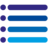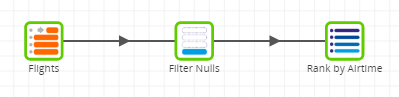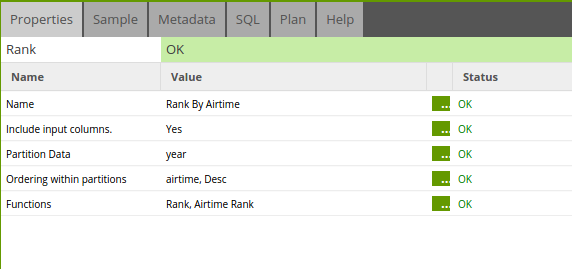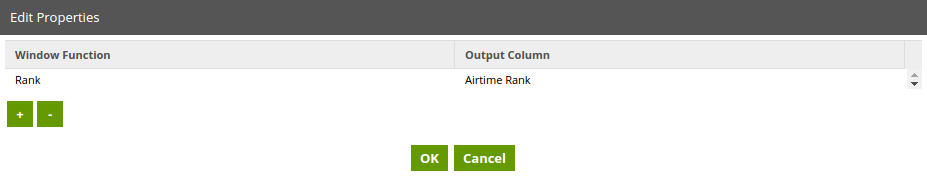• Matillion ETL v1.69
Rank
• Dark
Light

# Rank

• Dark
Light## Rank Component

Allows the user to determine the rank of a value in a group of values, output as a new column. The function used depends on the data warehouse as below:

## Snowflake Properties

Property Setting Description
Name Text A human-readable name for the component.
Include Input Columns Yes/No Defines whether the component passes all input columns into the output.
Partition Data List of Columns Defines how the input data is partitioned in order to perform the rank calculation. The calculation is then performed on each partition.
Ordering within partitions Input Column The input column name for sorting within the partitioned data. Note: you can drag to reorder
Ordering The order of the sorting: Ascending (Asc) or Descending (Desc)
Functions Window Function Rank - determines the rank of a value in a group of values.
Dense Rank - determines the rank of a value in a group of values. The Dense Rank function differs from rank in one respect: If two or more rows tie, there is no gap in the sequence of ranked values.
Cumulative Distribution - determines the cumulative distribution of a value within a window or partition.
Percent Rank - Calculates the percent rank of a given row.
Row Number - Determines the ordinal number of the current row within a group of rows, counting from 1.
Output Column The name of the output column that the window function will create.

## Redshift Properties

Property Setting Description
Name Text A human-readable name for the component.
Include Input Columns Yes/No Defines whether the component passes all input columns into the output.
Partition Data List of Columns Defines how the input data is partitioned in order to perform the rank calculation. The calculation is then performed on each partition.
Ordering within partitions Input Column The input column name for sorting within the partitioned data. Note: you can drag to reorder
Ordering The order of the sorting: Ascending (Asc) or Descending (Desc)
Functions Window Function Rank - determines the rank of a value in a group of values. More...
Dense Rank - determines the rank of a value in a group of values. The Dense Rank function differs from rank in one respect: If two or more rows tie, there is no gap in the sequence of ranked values. More...
Cumulative Distribution - determines the cumulative distribution of a value within a window or partition. More...
Percent Rank - Calculates the percent rank of a given row. More...
Row Number - Determines the ordinal number of the current row within a group of rows, counting from 1. More...
Output Column The name of the output column that the window function will create.

## BigQuery Properties

Property Setting Description
Name Text A human-readable name for the component.
Include Input Columns Yes/No Defines whether the component passes all input columns into the output.
Partition Data List of Columns Defines how the input data is partitioned in order to perform the rank calculation. The calculation is then performed on each partition.
Ordering within partitions Input Column The input column name for sorting within the partitioned data. Note: you can drag to reorder
Ordering The order of the sorting: Ascending (Asc) or Descending (Desc)
Functions Window Function Rank - determines the rank of a value in a group of values.
Dense Rank - determines the rank of a value in a group of values. The Dense Rank function differs from rank in one respect: If two or more rows tie, there is no gap in the sequence of ranked values.
Cumulative Distribution - determines the cumulative distribution of a value within a window or partition.
Percent Rank - Calculates the percent rank of a given row.
Row Number - Determines the ordinal number of the current row within a group of rows, counting from 1.
Output Column The name of the output column that the window function will create.

## Synapse Properties

Property Setting Description
Name Text A human-readable name for the component.
Include Input Columns Yes/No Defines whether the component passes all input columns into the output.
Partition Data List of Columns Defines how the input data is partitioned in order to perform the rank calculation. The calculation is then performed on each partition.
Ordering within partitions Input Column The input column name for sorting within the partitioned data. Note: you can drag to reorder
Ordering The order of the sorting: Ascending (Asc) or Descending (Desc)
Functions Window Function Rank - determines the rank of a value in a group of values.
Dense Rank - determines the rank of a value in a group of values. The Dense Rank function differs from rank in one respect: If two or more rows tie, there is no gap in the sequence of ranked values.
Cumulative Distribution - determines the cumulative distribution of a value within a window or partition.
Percent Rank - Calculates the percent rank of a given row.
Row Number - Determines the ordinal number of the current row within a group of rows, counting from 1.
Output Column The name of the output column that the window function will create.

## Delta Lake Properties

Property Setting Description
Name Text A human-readable name for the component.
Include Input Columns Select Defines whether the component passes all input columns into the output.
Partition Data Column Select Defines how the input data is partitioned in order to perform the rank calculation. The calculation is then performed on each partition.
Ordering within partitions Input Column The input column name for sorting within the partitioned data.
Note: you can drag to reorder.
Ordering The order of the sorting: Ascending (Asc) or Descending (Desc)
Functions Window Function Cumulative Distribution: determines the cumulative distribution of a value within a window or partition.
Dense Rank: determines the rank of a value in a group of values. The Dense Rank function differs from rank in one respect: If two or more rows tie, there is no gap in the sequence of ranked values.
Percent Rank: calculates the percent rank of a given row.
Rank: determines the rank of a value in a group of values.
Row Number: determines the ordinal number of the current row within a group of rows, counting from 1.
Output Column The name of the output column that the window function will create.

## Strategy

Snowflake: Generates a select statement with a window function in line using the OVER keyword. For more information, read Window Functions.

Amazon Redshift: Generates a select statement with a window function in line using the OVER keyword. For more information, read Window functions.

Azure Synapse: Generates a select statement with a window function in line using the OVER keyword. For more information, read Window functions.

Delta Lake on Databricks: Generates a select statement with a window function in line using the OVER keyword. For more information, read Window functions.

## Example

In this example we wish to determine the longest flight by airtime for a given year. Luckily, we have a table full of flight data already. The job is set up as below.The filter component is used to filter any null values from the data where airtime was not properly recorded. The remaining rows pass on to the Rank component that is set up as shown below.'Include Input Columns' is set to Yes so that all input columns are also output. The data is partitioned by year; in this case, this means that our output data will rank airtimes for the year 2000 followed by airtimes for the year 1999 and so on.

The 'Ordering within Partitions' property is the key to our ranking and is set to rank by airtime, descending.

Finally, the 'Rank' function is chosen and the new output column is called 'Airtime Rank'. This new column will show the rank of that particular row for that year.The output data can now be sampled from the Rank component and should reveal the longest airtime at the top of the sample.